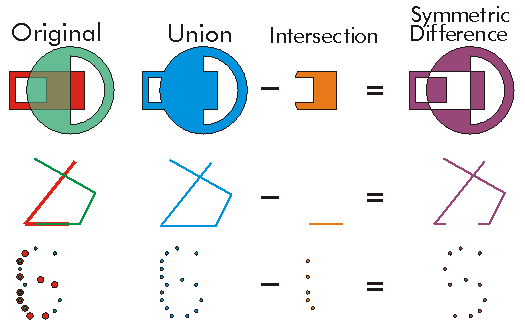This document is archived and information here might be outdated.  Recommended version.

ITopologicalOperator.Intersect Method (ArcObjects .NET 10.7 SDK)
 ArcObjects Help for .NET developers > ArcObjects Help for .NET developers > ArcObjects namespaces > Geometry > ESRI.ArcGIS.Geometry > Interfaces > IT > ITopologicalOperator Interface > ITopologicalOperator.Intersect Method
 ArcGIS Developer Help

# ITopologicalOperator.Intersect Method

Constructs the geometry that is the set-theoretic intersection of the input geometries. Use different resultDimension values to generate results of different dimensions.

```[Visual Basic .NET]
Public Function Intersect ( _
ByVal other As IGeometry, _
ByVal resultDimension As esriGeometryDimension _
) As IGeometry
```
```[C#]
public IGeometry Intersect (
IGeometry other,
esriGeometryDimension resultDimension
);
```
```[C++]
HRESULT Intersect(
IGeometry* other,
esriGeometryDimension resultDimension
);
```
```[C++]
Parameters
other
other is a parameter of type IGeometry*
resultDimension
resultDimension is a parameter of type esriGeometryDimension
```

#### Product Availability

Available with ArcGIS Engine, ArcGIS Desktop, and ArcGIS Server.

#### Description

When two geometries of the same dimension intersect with resultDimension the same as the input geometries, the output is a geometry containing only the regions of overlap between the original geometries.

#### Remarks

Intersection is basically an AND between input geometries.

ITopologicalOperator methods must be applied on high-level geometries only. High-Level geometries are point, multipoint, polyline and polygon. To use this method with low-level geometries such as segments (Line, Circular Arc, Elliptic Arc, B�zier Curve), paths or rings, they must be wrapped into high-level geometries types.

Note that when esriGeometryNoDimension is specified as resultDimension, the output will be in the dimension of the minimum dimension of the input geometries.

This method does not support GeometryBags.

Since ArcGIS 9.2, Intersect has a larger cost - it takes longer to run the method.  Therefore, it is a better approach to test if the two geometries are disjoint before calling Intersect.[C#]

//The following code shows to wrap a line segment into a polyline in C#

//Assume a line (line1 as ILine) is already created

object obj = Type.Missing;

ISegmentCollection segCollection = new PolylineClass() as ISegmentCollection;

segCollection.AddSegment((ISegment)line1, ref obj, ref obj);

//Set the spatial reference on the new polyline

//The spatial reference is not transfered automatically from the segments

IGeometry geom = segCollection as IGeometry;

geom.SpatialReference = spatialRef;

//Can now be used with ITopologicalOperator methods